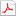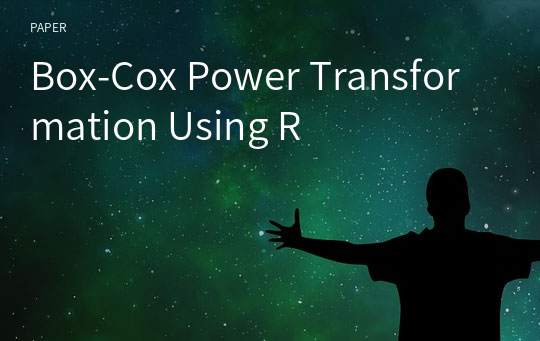# Box-Cox Power Transformation Using R

최초 등록일
2020.08.06
최종 저작일
2020.06
7페이지/어도비 PDF
가격
판매자(주)학술교육원
다운로드
장바구니

* 본 문서는 배포용으로 복사 및 편집이 불가합니다.

## 서지정보

발행기관 : 조선대학교 기초과학연구원 수록지정보 : 조선자연과학논문집 / 13권 / 2호 / 76 ~ 82 페이지
저자명 : Hoh Yoo Baek

## 영어 초록

If normality of an observed data is not a viable assumption, we can carry out normal-theory analyses by suitable transforming data. Power transformation by Box and Cox, one of the transformation methods, is derived the power which maximized the likelihood function. But it doesn't induces the closed form in mathematical analysis. In this paper, we compose some R the syntax of which is easier than other statistical packages for deriving the power with using numerical methods. Also, by using R, we show the transformed data approximately distributed the normal through Q-Q plot in univariate and bivariate cases with some examples. Finally, we present the value of a goodness-of-fit statistic(AD) and its p-value for normal distribution. In the similar procedure, this method can be extended to more than bivariate case.

없음

더보기 (2/7)

## 자료문의

제휴사는 별도로 자료문의를 받지 않고 있습니다.

## 판매자 정보학술논문 검색서비스를 제공하는 전문 판매자입니다.

본 학술논문은 (주)학술교육원과 각 학회간에 저작권계약이 체결된 것으로 AgentSoft가 제공 하고 있습니다.
본 저작물을 불법적으로 이용시는 법적인 제재가 가해질 수 있습니다.최근 본 자료더보기